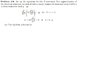# Query on indexing to determine coefficients

• bugatti79

#### bugatti79

Folks,
I am interested to know what the author is doing in the following

##\displaystyle B_{ij}=EL ij (L)^{(i+j-1)} \left[ \frac{(i-1)(j-1)}{i+j-3} -\frac{2(ij-1)}{i+j-2}+\frac{(i+1)(j+1)}{i+j-1}\right]##

he states that this expression is not valid for ##B_{ij}## when ##i=1## and ##j=1,2,...N##

...yet he goes on to actually calculate

##B_{11}=4EIL##, ##B_{1j}=B_{j1}=2EIL^j##, ##(j>1)##

I understand the the numerator in the first 2 terms inside the big brakets are both 0 when i=j=1 but we still yield a value from the third term...
Any insight will be appreciated
Regards

PS:I notice there is some editing problem with the 3 terms inside the big brackets. There should be a minus and plus separating the terms.

Folks,
I am interested to know what the author is doing in the following

##\displaystyle B_{ij}=EL ij (L)^{(i+j-1)} \left[ \frac{(i-1)(j-1)}{i+j-3} -\frac{2(ij-1)}{i+j-2}+\frac{(i+1)(j+1)}{i+j-1}\right]##

he states that this expression is not valid for ##B_{ij}## when ##i=1## and ##j=1,2,...N##
That's not at all obvious. The only restrictions I see are that
1. i + j ≠ 3 (would make the first denominator vanish)
2. i + j ≠ 2 (would make the second denominator vanish)
3. i + j ≠ 1 (would make the third denominator vanish)
...yet he goes on to actually calculate

##B_{11}=4EIL##, ##B_{1j}=B_{j1}=2EIL^j##, ##(j>1)##
I don't see how. With i = 1, j = 1, the second term in the brackets is 0/0.
I understand the the numerator in the first 2 terms inside the big brakets are both 0 when i=j=1 but we still yield a value from the third term...
Any insight will be appreciated
Regards

PS:I notice there is some editing problem with the 3 terms inside the big brackets. There should be a minus and plus separating the terms.

Could you give a link to where you found this question?

I don't see how. With i = 1, j = 1, the second term in the brackets is 0/0.

Are you saying because one of the terms is indeterminate then the whole equation is invalid and thus cannot be usedt o calcualte ##B_{ij}## for i=j=1?

Could you give a link to where you found this question?

See attached jpeg of question. The answer involves converting the DE into a weak form using a weight function w and splitting the differentiation between the weight function and the dependent variable u.
Would the choice of the approximation functions affect the outcome?

regards

#### Attachments

•Question.jpg
16.9 KB · Views: 283